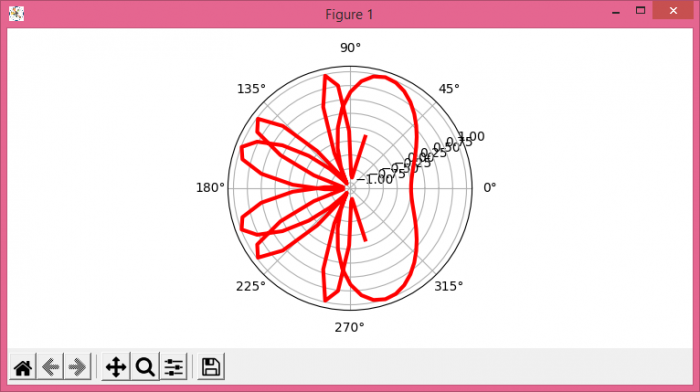# How to plot sine curve on polar axes using Matplotlib?

To plot the sine curve on polar axes, we can take the following steps −

• Set the figure size and adjust the padding between and around the subplots.
• Create a new figure or activate an existing figure using figure() method
• Add an '~.axes.Axes' to the figure as part of a subplot arrangement.
• Get x and y data points using numpy.
• Plot x and y data points using plot() method.
• To display the figure, use show() method.

## Example

import numpy as np
import matplotlib.pyplot as plt

plt.rcParams["figure.figsize"] = [7.50, 3.50]
plt.rcParams["figure.autolayout"] = True

fig = plt.figure()

x = np.linspace(-5, 5, 100)
y = np.sin(x**2)

ax.plot(x, y, color='red', lw=3)

plt.show()

## Output